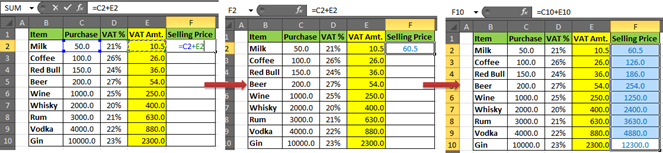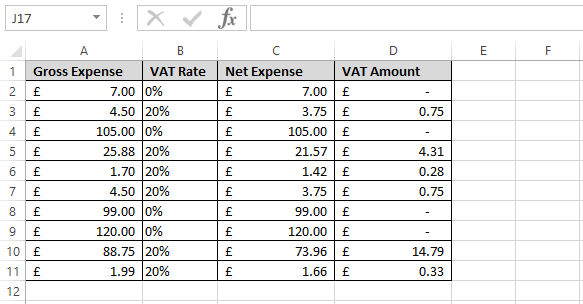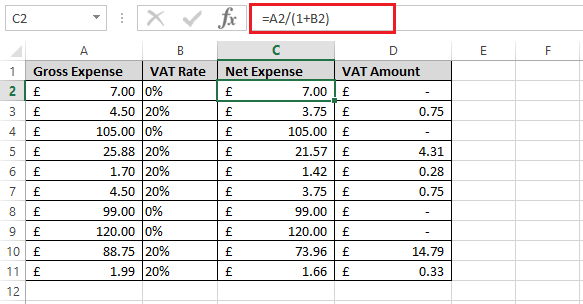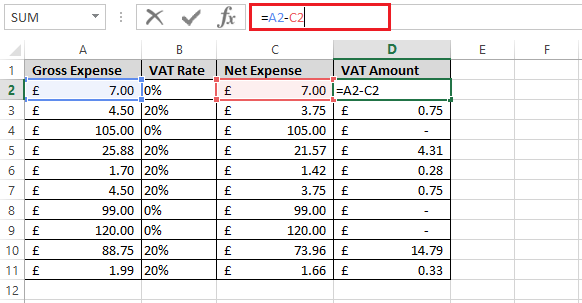# How to calculate VAT in Excel

Value-added tax (VAT) is also known as Goods and Service Tax (GST) in some counties. It is often a consumption tax levied on a product at each stage of the manufacturing process. I.e. from the initial steps of raw material and labor to the final steps of selling the product. The VAT is founded on consumption rather than income and is charged equally on the haves and haves, not on every purchase. To calculate the value-added tax amount on every stage that is paid, the amount of VAT on the latest stage is taken and subtracted from the VAT that already has been paid.

VAT calculations may also be done in Excel and are one of the staple business functions. Many different formulas can be used to calculate the VAT once you understand the basic principle. We are going to focus on;

• VAT Calculation in Excel

Method 1

The Formula that is Generic for VAT calculation in Excel is:

VAT = Purchase Price * %VAT

And for the selling price the Generic Formula for Calculating is:

Selling Price = Purchase + Taxes

Let's have an example of various Drinks. Assuming that Different %VAT is to various drink products. And we know the %VAT. It is possible to calculate the VAT and the selling price.

1. Let us prepare our table in an excel spreadsheet2. Calculating the VAT amount will be done by applying the formula

VAT = Purchase Price * %VAT

In which in our case is =D2*C2

This will give the VAT amount calculated and displayed for milk at E4. Drag down the formula to apply to E10.The % sign is required in the Vat percentage field. If you don't want to use the percent symbol, put your percentage with a "0" before it. Because excel translates "number percent" to "number/100."

3. Having the amount of VAT you can now calculate the selling price

We will apply the formula for selling price.

Selling Price = Purchase + Taxes

=C2+E2

In our case, we have taken VAT as the only tax that is applied to the product. We will then have the cost of the product as Purchase+VAT amt taxes.Method 2

Another formula we can use to calculate the VAT is

A2/ (1+B2)

A2-C2

Let us have the sample of a table with 4 columns ABCD. We will calculate the Net expense and net amount in C and D which is the VAT Amount. Column A is the Gross Expense which we already have and column B is the VAT Rate which is expressed as a percentage.Calculations of VAT are based on the assumption that the gross amount is always 120% of the net amount. This may be presented in a better way by dividing the gross by 20%. In our case, we only want to perform if the VAT rate is 20% in column B.

Because when we divide any number by 100% makes no difference since it 1. We can use the formula "1+VAT rate (Column B)" as shown below;Using this formula A2/1+B2, the expenses with a VAT rate of 0% tend to remain the same on net and gross totals. Any costs with a 20% VAT rate will show the net amount less the VAT.

Column D Displays the difference in Gross and Net amounts obtained by the formula =A2-C2 and thus we will obtain our VAT amount. Drag down to paste the formula.